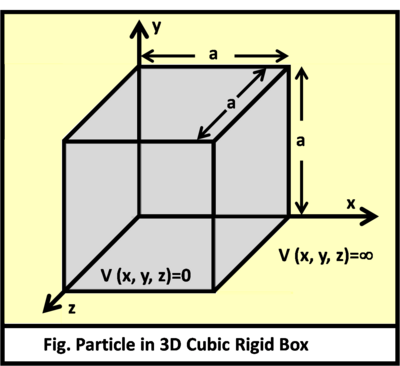# Discussion: 3D Quantum Particle-in-a-BoxHere we will solve the three dimensional particle in a box. To describe the system, we imagine a box with zero potential enclosed in dimensions $0, $0, $0. Outside the box is the region where the particle’s wavefunction does not exist. Hence, we let the potential outside the box be infinite (which means impossible to exist in physics).

$\begin{cases} 0\quad \quad \text{inside the box} \\ \infty \quad \text{outside the box} \end{cases}$

Boundary Conditions $\psi(0,y,z)=\psi(a,y,z)=0$ $\psi(x,0,z)=\psi(x,b,z)=0$ $\psi(x,y,c)=\psi(x,y,c)=0$

As always, wavefunctions are found by solving the Schrödinger equation.

$E \Psi (x,y,z) = -\frac{\hbar}{2m}\left[\frac{{\partial}^{2}}{\partial {x}^{2}}+\frac{{\partial}^{2}}{\partial {y}^{2}}+\frac{{\partial}^{2}}{\partial {y}^{2}}\right]\Psi (x,y,z) + V\Psi (x,y,z)$

We use the separation of variables to solve this partial differential equation (PDE). Consider the ansatz: $\Psi(x,y,z) = X(x)Y(y)Z(z)$

We also partition the total energy into its rightful components: $E={E}_{x} + {E}_{y} + {E}_{z}.$

Now we decompose the PDE into three ordinary differential equations (ODE):

$\frac{{d}^{2}X}{d{x}^{2}} + {k}_{x}^{2}X=0$

where ${k}_{x}=\sqrt{\frac{2m{E}_{x}}{{\hbar}^{2}}} =\frac{n \pi}{a}$ is the wavenumber, where $n$ is the mode.

For the $y$ and $z$ variables, we replace the above equation with $Y$ and $Z$. Likewise,

${k}_{y}=\sqrt{\frac{2m{E}_{y}}{{\hbar}^{2}}} =\frac{n \pi}{b}$ ${k}_{z}=\sqrt{\frac{2m{E}_{z}}{{\hbar}^{2}}} =\frac{n \pi}{c}.$

Solving the individual ODEs we find that

$X(x) = {A}_{x}sin({k}_{x}x)+ {B}_{x}cos({k}_{x}x)$ $Y(y) = {A}_{y}sin({k}_{y}y)+ {B}_{y}cos({k}_{y}y)$ $Z(z) = {A}_{z}sin({k}_{z}z)+ {B}_{z}cos({k}_{z}z).$

By the boundary conditions:

$X(0)=X(a)=0$ $Y(0)=Y(b)=0$ $Z(0)=Z(c)=0$

hence

${B}_{x}=0$ ${B}_{y}=0$ ${B}_{z}=0.$

We normalize the wavefunctions by performing the integral

$\int _{ 0 }^{ a }{ {\left| { { A }_{ x } } \right|} ^{2} } { sin }^{ 2 }\left( { k }_{ x }x \right) dx=1$

and applying the boundary condition $X(0)=X(a)=0$.

Thus ${A}_{x}=\sqrt{\frac{2}{a}}$.

Combine the solutions of all components to the ansatz yields

$\Psi (x,y,z) = \sqrt {\frac{8}{abc}}sin\left(\frac{n \pi}{a}x\right)sin\left(\frac{n \pi}{b}y\right)sin\left(\frac{n \pi}{c}z\right).$

Visit my set Lectures on Quantum Mechanics for more notes.

Check out my other notes at Proof, Disproof, and DerivationNote by Steven Zheng
6 years, 7 months ago

This discussion board is a place to discuss our Daily Challenges and the math and science related to those challenges. Explanations are more than just a solution — they should explain the steps and thinking strategies that you used to obtain the solution. Comments should further the discussion of math and science.

When posting on Brilliant:

• Use the emojis to react to an explanation, whether you're congratulating a job well done , or just really confused .
• Ask specific questions about the challenge or the steps in somebody's explanation. Well-posed questions can add a lot to the discussion, but posting "I don't understand!" doesn't help anyone.
• Try to contribute something new to the discussion, whether it is an extension, generalization or other idea related to the challenge.

MarkdownAppears as
*italics* or _italics_ italics
**bold** or __bold__ bold
- bulleted- list
• bulleted
• list
1. numbered2. list
1. numbered
2. list
Note: you must add a full line of space before and after lists for them to show up correctly
paragraph 1paragraph 2

paragraph 1

paragraph 2

[example link](https://brilliant.org)example link
> This is a quote
This is a quote
    # I indented these lines
# 4 spaces, and now they show
# up as a code block.

print "hello world"
# I indented these lines
# 4 spaces, and now they show
# up as a code block.

print "hello world"
MathAppears as
Remember to wrap math in $$ ... $$ or $ ... $ to ensure proper formatting.
2 \times 3 $2 \times 3$
2^{34} $2^{34}$
a_{i-1} $a_{i-1}$
\frac{2}{3} $\frac{2}{3}$
\sqrt{2} $\sqrt{2}$
\sum_{i=1}^3 $\sum_{i=1}^3$
\sin \theta $\sin \theta$
\boxed{123} $\boxed{123}$

Sort by:

thanksssssssssssssssssssssssssssssssssssssssss

- 6 years, 7 months ago

You're welcome!

- 6 years, 7 months ago

Elegant way to bring back those good ol' equations. Nice!

- 6 years, 7 months ago

Thank you!

- 6 years, 7 months ago

wow..wow....quantum mechanics..hummm...at first i thought it would be some KTOG problem....really inspired....nice note...please keep on posting

- 6 years, 6 months ago

What's a KTOG problem?

- 6 years, 6 months ago

Kinetic Theory Of Gases......

- 6 years, 6 months ago

ohhh. that makes sense. lol

- 6 years, 5 months ago